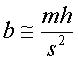Teaching Physics with the Physics Suite Edward F. Redish

Air Resistance 2: Estimating the effect

In the previous problem, we demonstrate that the force of air resistance on a sphere of radius R can plausibly be argued to have the formwhere the v with an arrow is the vector velocity and the v without is its magnitude (the speed). The density of the air, r, is about 1 kg/m3 --- 1/1000 that of water. The parameter C is a dimensionless constant.

If we drop a steel ball and a styrofoam ball from a height of s, the steel ball reaches the ground when the styrofoam ball is still a bit above the ground -- call the distance h. Estimate the air resistance coefficient C as follows:

(a) Assume the effect of air resistance on the steel sphere is negligible. Calculate approximately how how long the steel sphere takes to fall to the ground (t) and how fast it is travelling just before it hits (v). Express your answers in terms of s, g, and m.

(b) Since the steel and styrofoam were not very different, use vav, the average velocity of the steel ball during its fall to calculate an average air resistance force, Fav = -b vav2, acting on the styrofoam sphere during its fall. Express this force in terms of b, m (the mass of the styrofoam sphere), g, s, and h.

(c) The average velocity of the steel ball is vav,steel = s/t. The average velocity of the styrofoam sphere was vav,sty = (s-h)/t. Assume this difference is caused by the average air resistance force acting over the time t with our basic Newton's law formula:

Fav Dt = m Dv.

Use this to show that(d) A styrofoam ball of radius R = 5 cm and mass m = 50 gm, is dropped with a steel ball from a height of s = 2 m. When the steel ball hits, the styrofoam is about h = 10 cm above the ground. Calculate b (for the styrofoam sphere) and C (for any sphere).

Not finding what you wanted? Check the Site Map for more information.